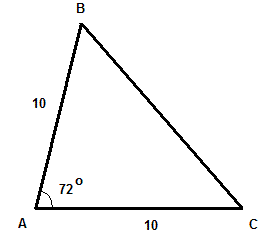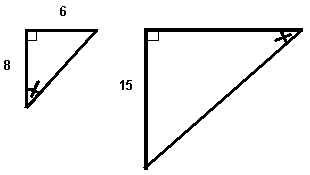The lengths of two sides of a triangle are 20 mm and 13 mm. Which of these lengths cannot represent the length of the third side. 35 mm 10 cm 20 mm 45 mm ABC is an isosceles triangle. Find the size of angle ABC.. The perimeter of an equilateral triangle is equal to 210 cm. What is the length of one side of this triangle? Find x so that the triangle shown below is a right triangle.. What will be the vertices of the triangle obtained by reflection on the a axis of the triangle defined by the vertices (1,2), (2,-3) and (4,-1)? The two triangles shown below are similar. Find the length of the hypotenuse of the larger triangle.. A 13 foot ladder is leaning against a vertical wall. The lowest point of the ladder is 4 feet from the wall. What is the height of the point where the ladder touches the wall? (round your answer to the nearest tenth of a foot). The length of the hypotenuse of a right triangle is 40 cm. The size of one of its angles is 45 degrees. What are the exact lengths of the other two sides of the triangle? Triangle ABC is an isosceles triangle. The length of the base is 20 meters and the corresponding height is 24 meters. Find the perimeter of ABC. (round your answer to the nearest tenth of a meter). A triangle has an area of 90 square cm. Find the length of the base if the corresponding base is 3 cm more then the height. The perimeter of a triangle is 74 inches. The length of the first side is twice the length of the second side. The third side is 4 inches more than than the first side. Find the length of each side of the triangle. Detemine the area of the triangle enclosed by the lines y = -4, x = 1 and y = -2x + 8: Show that the the triangle with vertices A(-1,6), B(2,6), C(2,2) is a right triangle and find its area. Answers to the Above Questions 35 mm, 10 cm = 100 mm and 45 mm cannot be the third side. 54 degrees 70 cm 1/2 (1,-2) , (2,3) and (4,1) length of hypotenuse is 25 units 12.4 feet 20 sqrt(2) cm 72 meters 15 cm 28 , 14 , 32 25 square units Calculate to find d(A,B) = 3 , d(BC) = 4 and d(A,C) = 5. Use the fact that d(A,B)2 + d(B,C)2 = d(A,C)2 and according to the converse of Pythagora's theorem the given triangle is a right triangle.